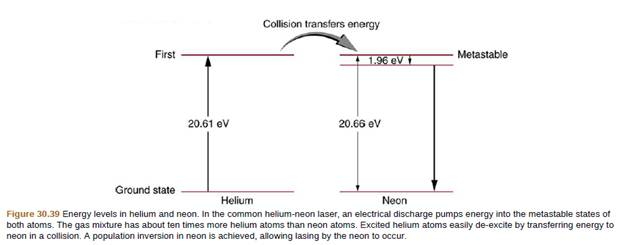Chapter 30, Problem 30PE

Chapter
Section
Textbook Problem

Figure 30.39 shows the energy-level diagram for neon. (a) Verity that the energy of the photon emitted when neon goes from its metastable state to the one immediately below is equal to 1.96 eV. (b) Show that the wavelength of this radiation is 633 nm. (c) What wavelength is emitted when the neon makes a direct transition to its ground state?To determine

(a)

To prove:

The energy released is equal to 1.96 eVwhen photon travels from meta-stable to immediate below.

Explanation

Given:

The energy in transition from ground state to first excited state = 20.61 eV = E1 Energy for the transition from first excited state to meta-stable state = 18.7 eV = E2

Formula used:

ΔE = change in energies = E1

To determine

(b)

To prove:

The wavelength of the photon is 633 nm.

To determine

(c)

The wavelength when neon makes a direct transition to ground state.

Still sussing out bartleby?

Check out a sample textbook solution.

See a sample solution

The Solution to Your Study Problems

Bartleby provides explanations to thousands of textbook problems written by our experts, many with advanced degrees!

Get Started

Find more solutions based on key concepts